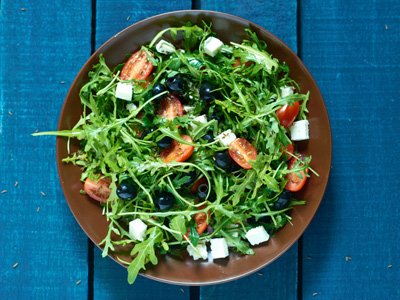Would a green salad be using thermal energy?

# Energy

This Science quiz is called 'Energy' and it has been written by teachers to help you if you are studying the subject at middle school. Playing educational quizzes is a fabulous way to learn if you are in the 6th, 7th or 8th grade - aged 11 to 14.

It costs only \$12.50 per month to play this quiz and over 3,500 others that help you with your school work. You can subscribe on the page at Join Us

What is energy? Energy, in short, is simply the ability by which people and things can work. It is the ability to change and/or move matter. The study of energy is performed by physicists. They study the energy’s forces and effects.

There are a number of different types of energy that include potential energy (or stored energy), kinetic energy (the motion of objects), thermal energy (caused when there is a sharp change in temperature), chemical energy (caused when a substance is transformed through a chemical reaction), nuclear energy (is energy associated with an atomic reaction), mechanical energy (is the sum of potential energy and kinetic energy) and electrical or electromagnetic energy (changes that are caused by the flow of charged ions or electrons through a current).

The energy in matter can cause the matter to change. For example, if you (the mass) stand still at the street corner waiting for the traffic light to turn red, you have potential energy. The potential energy is the ability to move forward across the street but by standing still, that potential energy is being stored.

Law of Conservation of Energy:

According to the Law of Conservation of Energy, energy is neither created nor destroyed. Rather, energy can only be converted from one form of energy to another form of energy.

1.
Looking at the following list of objects, which would be using chemical energy?
Pogo stick
Thermometer
Baseball bat
Ice cream
When a substance is transformed through a chemical reaction it is related to chemical energy. In this example, a thermometer that has mercury in it remains stationary until heated. When the thermometer is placed in or on a body, the body’s temperature is applied to the thermometer. The heat then causes the mercury in the thermometer to change, causing it to rise to give a reading. Answer (b) is correct
2.
Looking at the following list of objects, which would be using thermal energy?
Melting butter
Snow sled
As thermal energy is a change in matter brought about by a sharp increase or decrease in temperature, melting butter would signify that there was a sharp increase in temperature causing the butter to melt. Answer (a) is correct
3.
This is the form of energy an object has as it is moving.
Thermal energy
Kinetic energy
Potential energy
Stored energy
Kinetic energy is the form of energy an object has as it is moving. Answer (b) is correct
4.
Energy can only be ____________.
converted from one form of energy to another form of energy
electrical or mechanical
positive or negative
created and destroyed
Energy can only be converted from one form of energy to another form of energy. Answer (a) is correct
5.
Energy is never ______________.
balanced
without mass
stationary
created or destroyed
Energy is neither created nor destroyed. Answer (d) is correct
6.
Who studies energy?
Engineers
Doctors
Archeologists
Physicists
Physicists study energy, its sources and its effects. Answer (d) is correct
7.
If you are sitting down at a table, not moving, what kind of energy exists?
Stored energy
Thermal energy
Kinetic energy
Electrical energy
When an object (or mass) stays stationary, it contains energy but it is not currently being used. Therefore, the energy is being stored. Answer (a) is correct
8.
Energy is the ability to __________.
create
change or move matter
transform
reproduce
Energy is the ability to change and/or move matter. Answer (b) is correct
9.
Electrical or electromagnetic energy occurs when there is __________.
a sharp change in temperature
a change in a substance due to a chemical reaction
a flow of charge ions or electrons through a current
an atomic reaction
Electrical or electromagnetic energy occurs when there is a flow of charged ions or electrons through a current. Answer (c) is correct
10.
Looking at the following list of objects, which would be using kinetic energy?
A docked boat
A willow tree
Falling leaves
A sleeping cat
As kinetic energy is the form of energy an object has as it is moving, we must look at which object is moving. In this case, the docked boat, the willow tree and the sleeping cat are all stationary and not moving. However, the falling leaves are in a state of movement so they would be using kinetic energy. Answer (c) is correct
Author:  Christine G. Broome